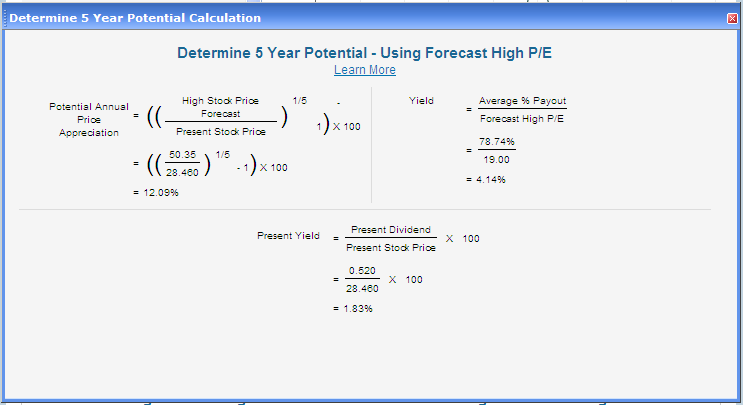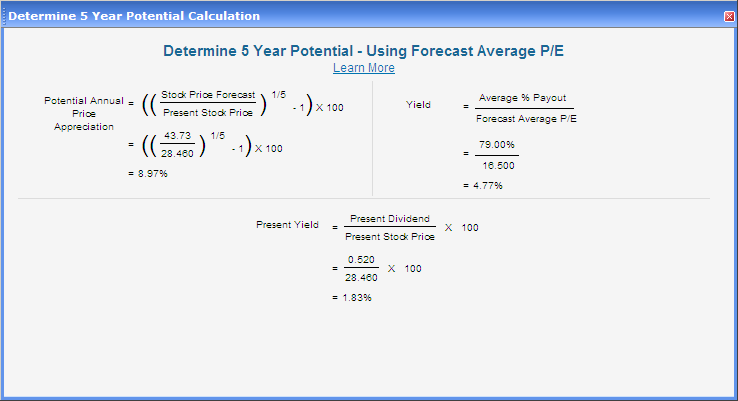Potential Return Calculations

The potential return that you might receive from investing in the company is calculated using your forecast high stock price and the current price of the stock. The formulas below take those two values and calculate the Compound Annual Growth Rate (CAGR) over 5 years.

The potential return labeled 'Using Forecast High P/E' uses your high stock price forecast from the study which was calculated by multiplying your high PE forecast times your 5 year high EPS forecast.The potential return labeled 'Using Forecast Average P/E' uses a high stock price which is calculated by multiplying the average of your high and low PE forecast times your 5 year high EPS forecast. This is typically a more conservative potential return than the 'Using Forecast High P/E' return.